Partial Likelihood Function for the Cox Model

Let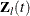denote the vector explanatory variables for the lth individual at time t. Let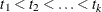denote the k distinct, ordered event times. Let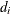denote the multiplicity of failures at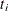; that is,is the size of the set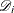of individuals that fail at. Let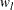be the weight associated with the lth individual. Using this notation, the likelihood functions used in PROC PHREG to estimateare described in the following sections.

Continuous Time Scale

Let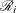denote the risk set just before the ith ordered event time. Let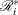denote the set of individuals whose event or censored times exceedor whose censored times are equal to.

Exact Likelihood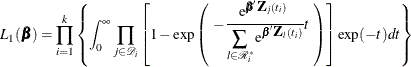Breslow Likelihood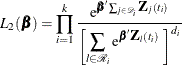Incorporating weights, the Breslow likelihood becomes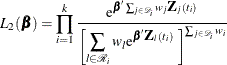Efron Likelihood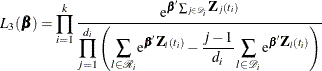Incorporating weights, the Efron likelihood becomes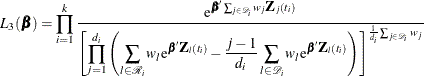Discrete Time Scale

Let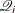denote the set of all subsets ofindividuals from the risk set. For each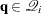,
is a-tuple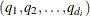of individuals who might have failed at.

Discrete Logistic Likelihood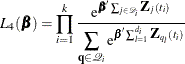The computation of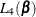and its derivatives is based on an adaptation of the recurrence algorithm of Gail, Lubin, and Rubinstein (1981) to the logarithmic scale. When there are no ties on the event times (that is,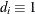), all four likelihood functions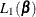,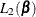,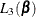, and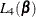reduce to the same expression. In a stratified analysis, the partial likelihood is the product of the partial likelihood functions for the individual strata.